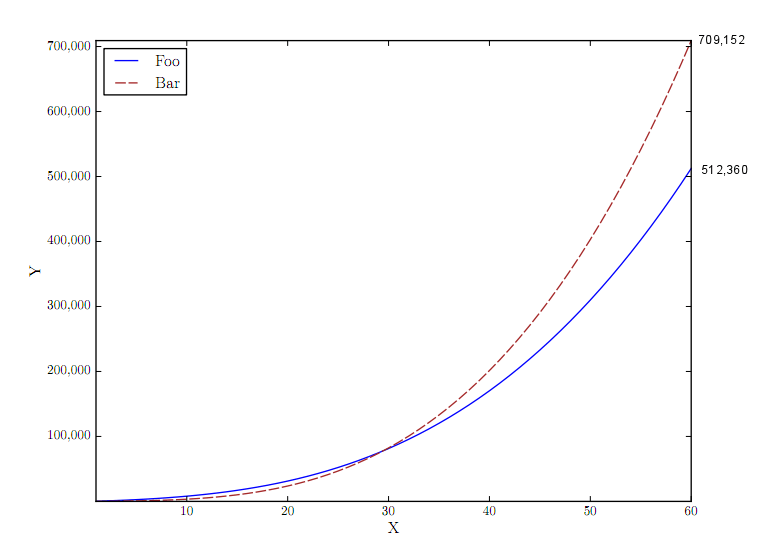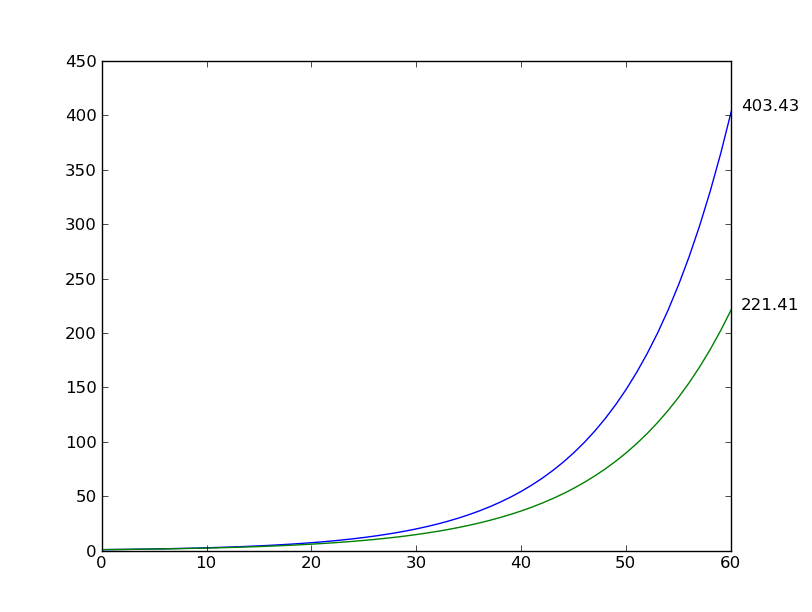# 关于python：用matplotlib显示每一行的最终y轴值

## Show the final y-axis value of each line with matplotlib123456789101112131415 import matplotlib.pyplot as plt import numpy as np x = np.arange(61).astype(np.float) y1 = np.exp(0.1 * x) y2 = np.exp(0.09 * x) plt.plot(x, y1) plt.plot(x, y2) for var in (y1, y2):     plt.annotate('%0.2f' % var.max(), xy=(1, var.max()), xytext=(8, 0),                  xycoords=('axes fraction', 'data'), textcoords='offset points') plt.show()1 pyplot.text(x, y, string, fontdict=None, withdash=False, **kwargs)

 123 second_axes = pyplot.twinx() # create the second axes, sharing x-axis second_axis.set_yticks([0.2,0.4]) # list of your y values pyplot.show() # update the figure

 123456 gbm = np.log(np.cumsum(np.random.randn(10000))+10000) plt.plot(gbm) plt.annotate('%0.2f' % gbm[-1], xy=(1, gbm[-1]), xytext=(8, 0),              xycoords=('axes fraction', 'data'), textcoords='offset points') plt.axhline(y=gbm[-1], color='y', linestyle='-.') plt.show()